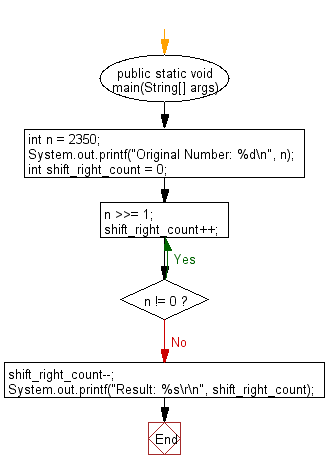﻿ Java programming exercises: Returns the largest integer but not larger than the base-2 logarithm of a specified integer - w3resource# Java Exercises: Returns the largest integer but not larger than the base-2 logarithm of a specified integer

## Java Basic: Exercise-156 with Solution

Write a Java program that returns the largest integer but not larger than the base-2 logarithm of a given integer.

Original Number: 2350
Result: 11

Sample Solution:

Java Code:

``````import java.util.Scanner;
public class Solution {

public static void main(String[] args) {
int n = 2350;
System.out.printf("Original Number: %d\n", n);
int shift_right_count = 0;
do {
n >>= 1;
shift_right_count++;
} while (n != 0);
shift_right_count--;
System.out.printf("Result: %s\r\n", shift_right_count);
}
}
```
```

Sample Output:

```Original Number: 2350
Result: 11
```

Flowchart:Java Code Editor:

What is the difficulty level of this exercise?

﻿

## Java: Tips of the Day

Parsing dates:

```import java.io.*;
import java.util.*;
import java.text.*;

String s = "2001/09/23 14:39";

SimpleDateFormat formatter = new SimpleDateFormat ("yyyy/MM/dd H:mm");
Date d = formatter.parse(s, new ParsePosition(0));
```